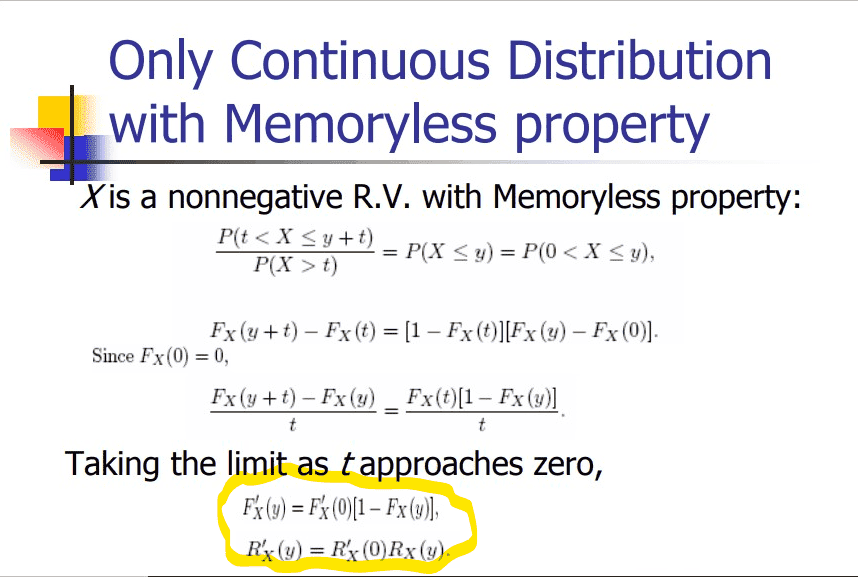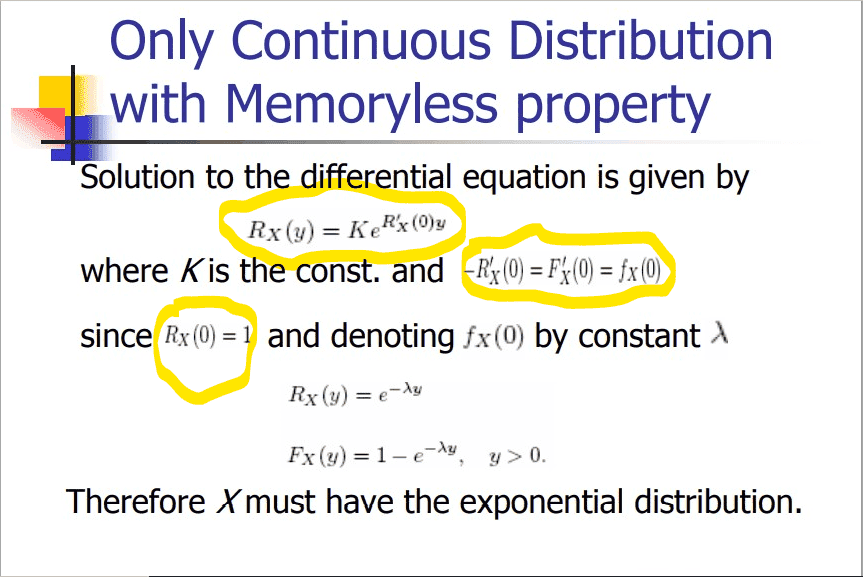# Exponential distribution question

• MHB
WMDhamnekar
MHB
Hi,I want to know how the highlighted steps are arrived at in the first page. What are $$R_X (y), R'_X (y),F'_X (0) ?$$How $$R_X (0) = 1 ?$$ Solution to differential equation should be $$R_X (y)=K*e^{\int{R'_X (0) dx}}$$ But it is different. How is that?

What is $-R'_X (0)=F'_X(0)=f_X(0)$ I know derivative of CDF is PDF, but in this case it is somewhat difficult to understand.

If any member of MHB knows how to satisfy my queries correctly, may reply to this question:unsure:

Homework Helper
MHB
Hey Dhamnekar Winod,

In the first step they apply:
$$\lim_{t\to 0} \frac{F_X(t)[1-F_X(y)]}{t} = \lim_{t\to 0} \frac{F_X(t)-F_X(0)}{t}\cdot [1-F_X(y)] = F_X'(0)\cdot [1-F_X(y)]$$

Apparently they defined $R_X(y)=1-F_X(y)$ as an intermediate step to solve the differential equation.
It follows that $R_X'(y)=-F_X'(y)$ and $R_X(0)=1$.
The corresponding equation then follows from the previous equation.

The solution of the differential equation is indeed:
$$R_X (y)=K\cdot e^{\displaystyle\int_0^y{R'_X (0) dx}} = K\cdot e^{\displaystyle\big[R'_X (0) x\big]_0^y} = K\cdot e^{R'_X (0) y}$$